• 基于CNN的鸢尾花分类器

千次阅读 2020-07-27 11:23:10
基于CNN鸢尾花分类鸢尾花为结构化数据，包括四个特征，三个标签 本次使用CNN来进行分类 废话不多说，请看代码 #导入所要用到的包 from sklearn import datasets from keras.layers import * import ...

基于CNN的鸢尾花分类器

鸢尾花为结构化数据，包括四个特征，三个标签

本次使用CNN来进行分类

废话不多说，请看代码

#导入所要用到的包

from sklearn import datasets
from keras.layers import *
import keras.backend as K
from keras import Model
from keras.utils import plot_model,to_categorical
import pandas as pd
import numpy as np
from keras.utils import plot_model
from sklearn.utils import shuffle
import matplotlib.pyplot as plt

#划分特征和标签

X = np.array(iris['data'])
Y = np.array(iris['target'])

fea_cnt = len(X) #特征个数
numb = max(Y)+1 # 分类类别个数

Y = np.array([to_categorical(y,numb,dtype='int32') for y in Y])

#构建模型，二维卷积
def build_model(fea_cnt,numb):
K.clear_session() #清除之前的模型，省得压满内存
inputs = Input(shape=(fea_cnt,), dtype='float32')
embds = Dense(64 ,activation='selu')(inputs)
embds = Reshape((8,8,1))(embds)
embds = Flatten()(embds)
embds = Dense(64,activation='selu')(embds)
embds = Dense(32,activation='selu')(embds)
outputs = Dense(numb,activation='softmax')(embds)

model = Model(inputs=inputs, outputs=outputs)
#model.summary()
return model

#数据集划分，20%的数据为测试集

model = build_model(fea_cnt,numb)
X,Y = shuffle(X,Y, random_state=1337) #打乱顺序进行分隔
validation_split=0.2

train_X = X[:int(len(X)*(1-validation_split))]
train_Y = Y[:int(len(Y)*(1-validation_split))]
test_X = X[int(len(X)*(1-validation_split)):]
test_Y = Y[int(len(Y)*(1-validation_split)):]

#训练模型
epochs = 12
batch_size = 4
model.fit(train_X ,train_Y
,batch_size = batch_size
,epochs = epochs
,validation_data= [test_X,test_Y]
)

plot_model(model, to_file='./model.png',show_shapes=True,dpi=300) #输出框架图
score = model.evaluate(test_X, test_Y)

#打印准确度与损失值

print('Test loss:', score)
print('Test accuracy:', score)

展开全文深度学习
• 利用神经网络对鸢尾花数据集分类 详细实现代码请见： 一.简介 一个人工神经元网络是由一个多层神经元结构组成，每一层神经元拥有输入（它的输入是前一层神经元的输出）和输出，我们把神经元和与之对应的神经元之间的...

利用神经网络对鸢尾花数据集分类

一.简介
一个人工神经元网络是由一个多层神经元结构组成，每一层神经元拥有输入（它的输入是前一层神经元的输出）和输出，我们把神经元和与之对应的神经元之间的连线用生物学的名称，叫做突触，在数学模型中每个突触有一个加权数值，称做权重，此时第i层上的某个神经元所得到的输出等于每一个权重乘以第i-1层上对应的神经元的输出之和，最后再通过激活函数来对输出进行量化，在与阈值相比较判断是否属于某一类。
本文主要对BP神经网络进行一些粗浅的研究，对神经网络的原理进行了解后，尝试编写基于matlab的BP神经网络代码，然后进行实验。主要采取matlab中自带的数据集：鸢尾花数据集，有三个类别且每个类别具有4个属性，采用K-fold（交叉验证）对数据集进行划分，获取训练数据和测试数据，使用训练集进行训练，得出感知器输出方程的各个权重，接下来对测试集进行测试，最终与原标签进行比较，计算准确率。再调用matlab中的神经网络包对测试集进行测试，得出结果与编写的进行比较。

二.问题描述与数据描述
（一）问题描述
通过本实验加深对神经网络的理解，实现使用BP神经网络对鸢尾花数据集进行分类。

（二）数据描述
matlab中有自带的鸢尾花数据集，且都是已经处理好成mat格式，故只要直接导入便可。它是很常用的一个数据集，鸢尾花有三个分类，分别是山鸢尾（Iris-setosa）、变色鸢尾（Iris-versicolor）和维吉尼亚鸢尾（Iris-virginica）。而且只有四个属性，分别是花萼长度、花萼宽度、花瓣长度、花瓣宽度。总共150条数据。
三.如何解决问题
（一）模型假设
将鸢尾花的属性以坐标形式表示，建立以下支持BP神经网络模型：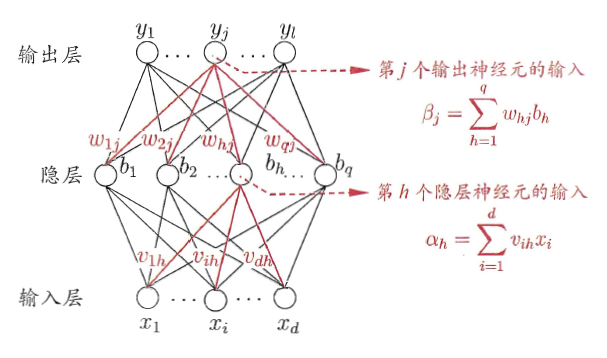1.如图所示，该神经网络一共有三层，输入层、隐藏层与输出层，输入的样本为x1、x2……xd（d=150），输出的向量为y1 y2……yl（l=3），隐藏层神经元数目为q。
2.其中输出层第j个神经元的阈值用θj表示，隐层第h个神经元的阙值用γh表示，输入层第i个神经元与隐层第h个神经元之间的连接权为vih，隐层第h个神经元与输出层第j个神经元之间的连接权为whj，bh为隐藏层第h个神经元的输出。选择sigmoid函数为激活函数。记隐层第h个神经元接收到的输入与输出层第j个神经元接收到的输入分别为：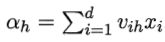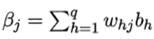3.对训练样本（xk，yk），神经网络的输出和均方误差分别为：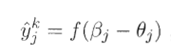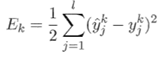4.上面的神经网络中有（d+1+1）q+l个参数需确定：输入层到隐层的d×q个权值、隐层到输出层的q×l个权值、q个隐层神经元的阈值、l个输出层神经元的阈值.BP是一个迭代学习算法，在迭代的每一轮中采用广义的感知机学习规则对参数进行更新估计，任意参数v的更新估计式为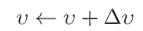使用梯度下降法，以目标的负梯度方向对参数进行调整。对Ek，给定学习率η，有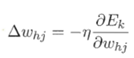注意到whj先影响到第j个输出层神经元的输出值βj，再影响到最终输出值，最后影响到Ek，所以有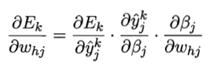由βj的定义有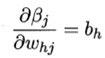Sigmoid函数的一个性质有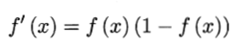由公式1、2得
公式10
再将公式8、10带入公式7，再代入公式6得到whj的更新公式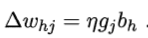同样可以得到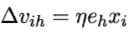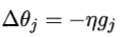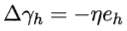其中eh为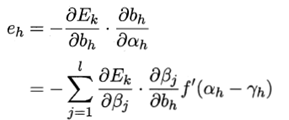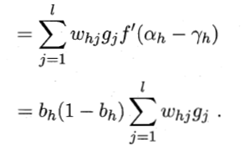5.总结
BP算法的工作流程：对每个训练样例，BP算法执行以下操作：
（1）先将输入示例提供给输入层神经元，然后逐层将信号前传，直到产生输出层的结果；
（2）然后计算输出层的误差（第4-5行），
（3）再将误差逆向传播至隐层神经元（第6行），
（4）最后根据隐层神经元的误差来对连接权和阈值进行调整（第7行该迭代过程循环进行，直到达到某些停止条件为止。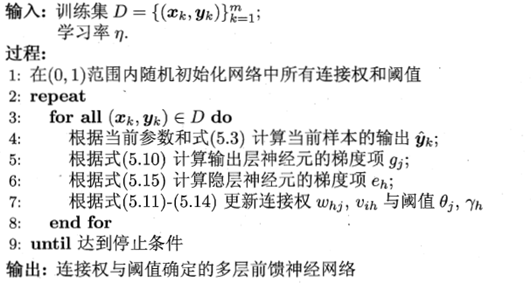（二）实现代码步骤
1.导入数据集
2.数据预处理
（1）转化标签
matlab自带的鸢尾花数据集的标签为setosa、versicolo和virginica，在建立神经网络的时候，要将其转化为一个3维的向量。如山鸢尾的标签为[1 0 0]、变色鸢尾的标签为[0 1 0]和维吉尼亚鸢尾的标签为[0 0 1]。
（2）数据归一化
将数据集的所有数据都归一化到[0,1]区间，以便提高模型的收敛速度与精度。
（3）划分训练集与测试集
交叉验证来选取训练集与测试集，将原始数据分成5组(K-Fold)，将每个子集数据分别做一次验证集，其余的4组子集数据作为训练集，这样会得到5个模型。这5个模型分别在验证集中评估结果，最后的结果加和平均就得到最终结果。交叉验证有效利用了有限的数据，使得评估结果能够尽可能接近模型在测试集上的表现。流程如下：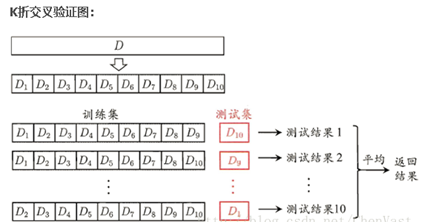本实验将数据集分为五组，每次选取四组为训练集，其他一组为测试集。对鸢尾花数据集进行五次训练，最终将得到五个结果，选取其平均值为最终结果。
（4）建立BP神经网络
根据模型假设中的算法设计编写参数更新代码(使用递归)，对训练集进行训练，得出最终的神经网络模型。
（5）测试准确率
对得到的模型进行检验，使用测试集进行仿真训练。
（6）调整模型
进行多次实验，对模型进行微调，如隐藏神经元数目、迭代最大次数、学习率。
（7）与内置包进行比较
调用神经网络包对数据进行训练测试，得出准确率，并进行比较。

四．数值实验
（一）分析结果
1.编程实现神经网络结果
第一次训练的准确率为：96.67%
第二次训练的准确率为：100%
第三次训练的准确率为：100%
第四次训练的准确率为：96.67%
第五次训练的准确率为：90%
平均准确率为：96.67%
2.调用神经网络包结果
第一次训练的准确率为：100%
第二次训练的准确率为：100%
第三次训练的准确率为：93.33%
第四次训练的准确率为：96.67%
第五次训练的准确率为：93.33%
平均准确率为：96.67%

五.总结
由于使用k-折交叉运算的方法进行多次实验，所以以最终平均准确率为标准，自定义BP神经网络的结果与调包使用的结果一致，都为96.67%，而从每一次的训练结果上看，也都保持在90%以上，都能够实现较好的分类。

六.参考文献与网站
机器学习导论
机器学习_周志华
K-Fold 交叉验证 (Cross-Validation)的理解与应用：https://www.cnblogs.com/xiaosongshine/p/10557891.html
matlab 中“newff” 函数的参数设置:
https://blog.csdn.net/weixin_30681121/article/details/99843850
利用BP神经网络分类iris数据集:
https://blog.csdn.net/lovefreewind/article/details/42679243
 matlab详解newff(前馈反向传播网络):
https://blog.csdn.net/xiaotao_1/article/details/79078729
 matlab神经网络不常见问题:
https://blog.csdn.net/weixin_42296976/article/details/81252809
 支持向量机与神经网络的区别:

展开全文神经网络 机器学习 算法 人工智能
• 1.1D-CNN from sklearn.model_selection import train_test_split from sklearn.datasets import load_iris from sklearn.preprocessing import LabelEncoder import keras from keras.models import Sequential ...

1.1D-CNN

from sklearn.model_selection import train_test_split
from sklearn.preprocessing import LabelEncoder

import keras
from keras.models import Sequential
from keras.layers import Dense, Flatten, Conv1D, MaxPool1D, Dropout

import matplotlib.pyplot as plt
import pandas as pd

x_train, x_test, y_train, y_test = train_test_split(iris.data, iris.target, test_size=0.33, random_state=1)

#  number of label classes
num_classes = len(pd.Series(iris.target).unique())
# print(num_classes)

#  label's label encoding
encoder = LabelEncoder()
y_train = encoder.fit_transform(y_train)
y_test = encoder.fit_transform(y_test)

# label's one-hot encoding
y_train = keras.utils.to_categorical(y_train, num_classes)
y_test = keras.utils.to_categorical(y_test, num_classes)

# reshape 2D to 3D ->  x_train.reshape(num_of_examples,num_of_features,num_of_signals)
x_train = x_train.reshape(x_train.shape, x_train.shape, 1)
x_test = x_test.reshape(x_test.shape, x_test.shape, 1)

# build CNN model
model = Sequential()
model.add(Conv1D(64, 2, input_shape=(4, 1), activation='relu'))  # convolution

# model compile
model.compile(loss=keras.losses.categorical_crossentropy,
metrics=['accuracy'])
model.summary()

# model training
batch_size = 1
epochs = 1000
model = model.fit(x_train, y_train,
batch_size=batch_size,
epochs=epochs,
verbose=2,
validation_data=(x_test, y_test))

# draw loss
plt.plot(model.history['loss'])
plt.plot(model.history['val_loss'])
plt.title('model train vs validation loss')
plt.ylabel('loss')
plt.xlabel('epoch')
plt.legend(['train', 'validation'], loc='upper right')
plt.show()

2.DNN

from sklearn.model_selection import train_test_split
from sklearn.preprocessing import LabelEncoder

import keras
from keras.models import Sequential
from keras.layers import Dense
import pandas as pd

import matplotlib.pyplot as plt

x_train, x_test, y_train, y_test = train_test_split(iris.data, iris.target, test_size=0.33, random_state=1)

#  number of label classes
num_classes = len(pd.Series(iris.target).unique())
# print(num_classes)

#  label's label encoding
encoder = LabelEncoder()
y_train = encoder.fit_transform(y_train)
y_test = encoder.fit_transform(y_test)

# label's one-hot encoding
y_train = keras.utils.to_categorical(y_train, num_classes)
y_test = keras.utils.to_categorical(y_test, num_classes)

model = Sequential()

# model compile
model.compile(loss=keras.losses.categorical_crossentropy,
metrics=['accuracy'])
model.summary()

# model training
batch_size = 1
epochs = 100
model = model.fit(x_train, y_train,
batch_size=batch_size,
epochs=epochs,
verbose=2,
validation_data=(x_test, y_test))

# draw loss
plt.plot(model.history['loss'])
plt.plot(model.history['val_loss'])
plt.title('model train vs validation loss')
plt.ylabel('loss')
plt.xlabel('epoch')
plt.legend(['train', 'validation'], loc='upper right')
plt.show()

3.1D-CNN accuracy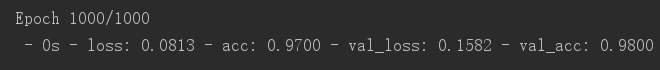4.DNN accuracy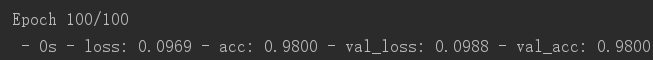展开全文神经网络 卷积神经网络 iris 分类算法
• CNN一般用来做图像数据处理，比较少见用于做普通数据预估。写了3个例子分享给大家参考参考。环境python37
• 1.首先导入鸢尾花数据 import numpy as np import pandas as pd import matplotlib.pyplot as plt dataset = pd.read_csv('Iris.csv') 2.使用seaborn对数据进行观察 import seaborn as sns sns.pairplot(dataset...

1.首先导入鸢尾花的数据

import numpy as np
import pandas as pd
import matplotlib.pyplot as plt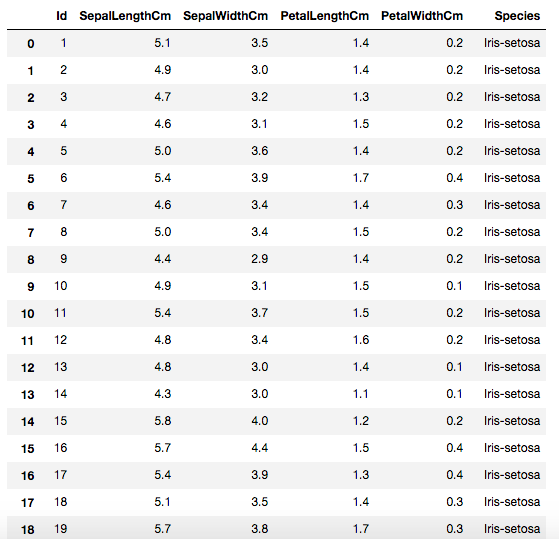2.使用seaborn对数据进行观察

import seaborn as sns
sns.pairplot(dataset.iloc[:, 1:6], hue='Species')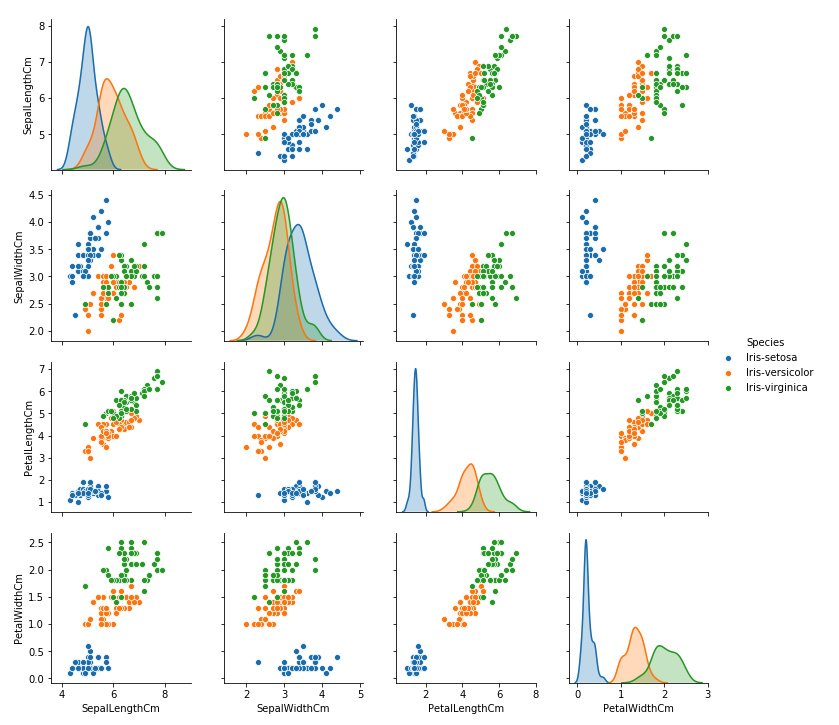3.生成测试数据

将前4列与第5列分别抽离成np array

X = dataset.iloc[:, 1:5].values
y = dataset.iloc[:,5].values

此时X是这样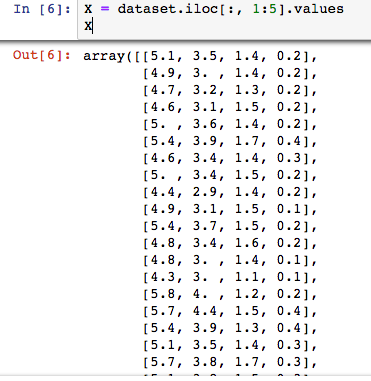但是y还是字符串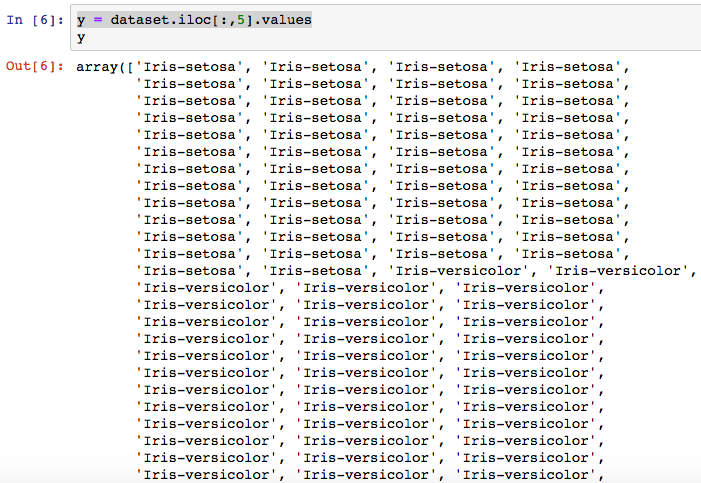所以我们要将y字符串数组转换成整数数组，在这里我们可以使用sklearn的LabelEncoder库

from sklearn.preprocessing import LabelEncoder
encoder = LabelEncoder()
y1 = encoder.fit_transform(y)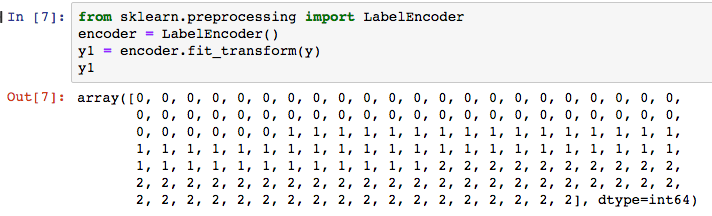最后将y1转成神经网络需要的数组结构

Y = pd.get_dummies(y1).values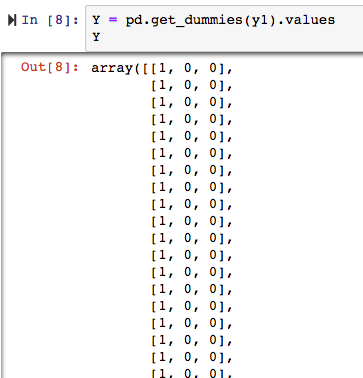4.将训练数据与测试数据做分割

from sklearn.model_selection import train_test_split
X_train, X_test, y_train, y_test = train_test_split(X, Y, test_size=0.2, random_state=0)

5.创建神经网络模型

使用Sequential创建神经网络模型
模型一共4层
损失函数使用‘categorical_crossentropy’（比较适用于3种以上的分类的情况）
指定 metrics=[‘accuracy’]，会在训练结束后计算训练数据在模型上的准确率

from keras.models import Sequential
from keras.layers import Dense

model = Sequential()

model.summary()

6.训练模型

指定epochs=100，训练数据会在模型中训练100次

model.fit(X_train, y_train, epochs=100)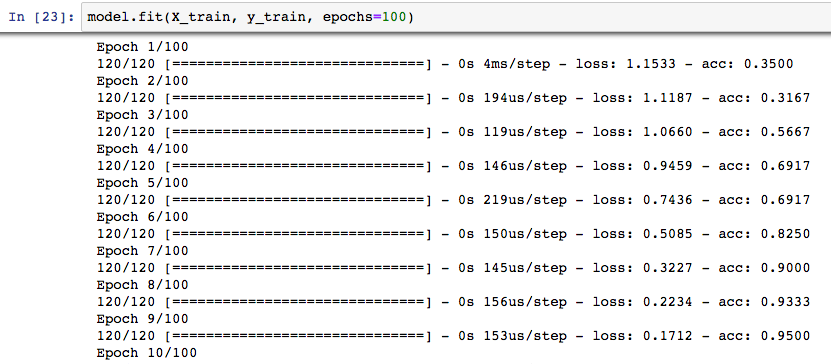7.使用模型进行预测

y_pred = model.predict(X_test)

打印结果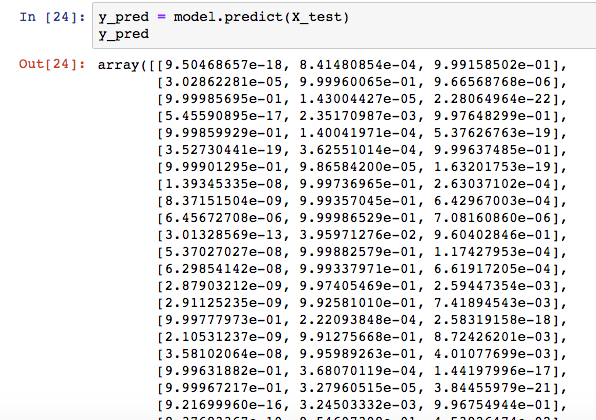浮点类型的数据不方便理解，所以使用np.argmax将数据转为整数数组

y_pred_class = np.argmax(y_pred, axis=1)  //其实就是记录每个数组中值最大的数的index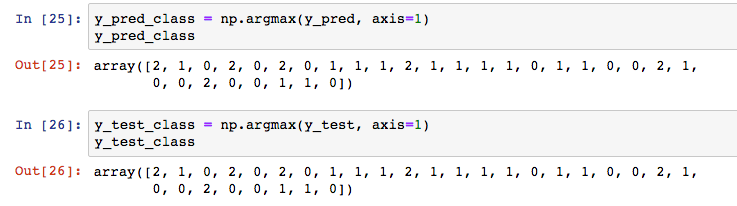以上就是得到预测数据的全过程，当然最后我们还是需要一个更直观的方式来评估模型准确率

6.模型评估

from sklearn.metrics import classification_report
report = classification_report(y_test_class, y_pred_class)
print(report)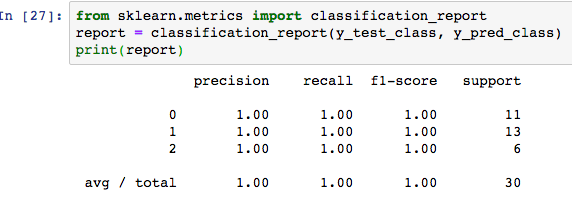precision表示测试的数据是否都预测准确
recall表示需要查的数据是否都查到了
f1=2*(precision*recall)/(precision+recall)
support表示测试数据中属于各个分类的测试数据各有多少个
由此可观察到，此时测试数据在模型上的准确率达到了100%

展开全文深度神经网络
• kNN处理鸢尾花数据集

千次阅读 2019-11-08 16:13:06
这里以鸢尾花数据集为例，讨论分类问题中的 kNN 的思想。 鸢尾花数据集内包含 3 类共 150 条记录，每类各 50 个数据，每条记录都有 4 项特征：花萼长度（sepal length）、花萼宽度（sepal width）、花瓣长度（petal...kNN sklearn
• 一.iris数据集简介 iris数据集的中文名是安德森鸢尾花卉数据集，英文全称是Anderson...通俗地说，iris数据集是用来给分类数据集，每个样本包含了花萼长度、花萼宽度、花瓣长度、花瓣宽度四个特征（前4列），...
• 数据集介绍 Iris也称鸢尾花卉数据集，是一类多重变量分析的数据集数据集包含150个数据样本，分为3类，每类50个数据，每个数据包含4个属性。...用CNN分类Iris数据集 from sklearn.datasets import load_ir...Iris数据集
• iris 鸢尾花数据集

千次阅读 2018-03-19 10:25:27
这是个鸢尾花的三分类数据集, 太经典了. 这里描述一下. tensorflow 的入门教程也有描述, 见 参考. 它的数据集要在运行代码时从网上下载, 因为 Great Fire Wall, 下载会失败. 所以介绍下 sci-kit learn 自带的...
• Iris数据集是常用的分类实验数据集，由Fisher, 1936收集整理。Iris也称鸢尾花卉数据集，是一类多重变量分析的数据集 拿到数据 scikit-learn数据集API介绍 sklearn中获取数据集的方法 sklearn.datasets 加载获取流行...
• 文章目录基于鸢尾花（iris）数据集在多分类上进行训练和预测1、数据可视化和特征分析2、分录指标评价计算3、利用混淆矩阵对于结果进行可视化4、总结 想要看更加舒服的排版、更加准时的推送 关注公众号“不太灵光...
• 通常人们根据生活经验，量取鸢尾花的花萼长、宽，花瓣长、宽，并且依据数据间的关系判断鸢尾花的类别。比如，花萼长>花萼宽且花瓣长为花瓣宽的两倍以上时为杂色鸢尾花（1）。(本文将鸢尾花分为三类，分别为狗尾草...tensorflow 深度学习 python
• pytorch解决鸢尾花分类

千次阅读 2018-12-12 16:30:00
半年前用numpy写了个鸢尾花分类200行。。每一步计算都是手写的 python构建bp神经网络_鸢尾花分类 现在用pytorch简单写一遍，pytorch语法解释请看上一篇pytorch搭建简单网络 1 import pandas as pd 2 import ...
• [PyTorch小试牛刀]实战二·实现逻辑回归对鸢尾花进行分类 代码 import numpy as np import pandas as pd import matplotlib.pyplot as plt import torch as t from torch.autograd import Variable as var file_...
• ) ''' 基于MLP（Multi-layer Perceptron）多层感知器的softmax多分类 ''' model = Sequential() # Dense(128) is a fully-connected layer with 128 hidden units. # in the first layer, you must specify the ...深度学习 神经网络
• keras实现糖尿病预测，鸢尾花数据集分类是一个多分类问题，至此关于数据的二分类和多分类问题都已经整理完毕。这也是一个小的技巧，当遇到一个关于数据的二分类或者是多分类问题的时候可以先找找一些相关的经典的...机器学习 多分类
• # 神经网络实现鸢尾花分类 import tensorflow as tf import numpy as np # 读取数据集 from sklearn.datasets import load_iris iris = load_iris() x_data = iris['data'] y_data = iris['target'] # 使数据集...tensorflow 深度学习 神经网络
• 对于Iris数据集，使用模糊聚类及模糊推理系统其进行分类模糊系统 模糊聚类 MATLAB 机器学习 鸢尾花Iris
• 我们可以根据花萼长，花萼宽，花瓣长，花瓣宽这四个特征值对鸢尾花进行分类。 神经网络设计 神经网络结构：单层前馈型神经网络 激活函数：softmax函数 损失函数：交叉熵损失函数 Y=WX+B 共有四个特征值，结果分为三...分类 tensorflow 深度学习
• 鸢尾花识别

千次阅读 多人点赞 2020-04-13 21:19:11
鸢尾花数据集是一类多重变量分析的数据集。通过花萼长度，花萼宽度，花瓣长度，花瓣宽度4个属性预测鸢尾花卉属于(Setosa，Versicolour，Virginica)三个种类中的哪一类(其中分别用0，1，2代替)。 数据集中部分数据与...机器学习 深度学习 数据挖掘
• 鸢尾花分类——神经网络详解

万次阅读 多人点赞 2016-04-26 19:16:34
设计合理地神经网络通过系统输入输出样本进行自动学习，能够以任意精度逼近任何复杂的非线性映射。神经网络的这一优点能使其可以作为多维非线性函数的通用数学模型。该模型的表达式非解析的，输入输出数据之间的...
• 适用于有着在机器学习/或者深度学习有着理论基础，拥有一定python编程基础但是神经网络实践缺少经验的coder，通过阅读并且自己完成本篇博客中的代码小白也能学会如何利用神经网络来实现简单的分类任务。...tensorflow 神经网络 深度学习 鸢尾花分类
• 刚刚学习了pytorch框架，尝试着使用框架...2. 完成建立鸢尾花分类模型（可尝试使用 K 近邻、决策树、SVM 等不同算法）（这里使用的神经网络） 3. 建立的模型进行评估 4. 画出loss曲线、ROC曲线，accuracy曲线 一.pytorch 分类 神经网络
• 本blog为观看MOOC视频与网易云课堂所做的笔记 ...用CNN做图像分类的时候，为什么要一批一批地输入数据样本数据的处理方法： 传统的梯度下降法：用全部样本计算迭代时的梯度 随机梯度下降法(在线梯度.python 机器学习 深度学习 神经网络 人工智能
• tensorflow2.0 python3.7 环境下利用神经网络实现鸢尾花分裂 数据集处理 iris = load_iris() x_data = iris.get('data')#返回iris数据集所有特征 y_data = iris.get('target')#返回iris数据集的所有标签 #数据集...python tensorflow 神经网络
• Keras 函数式编程实现鸢尾花分类Keras 函数式编程数据准备导入包导入数据集数据处理构建模型输入层中间层输出层构建模型损失函数和梯度更新保存模型 Keras 函数式编程 Keras 函数式编程定义复杂模型，可以参考 c++ ...tensorflow 深度学习 神经网络
• 文章目录一、说明二、题目三、实践部分四、源代码 一、说明 我是在jupyter完成的，然后导出成markdown格式，ipynb文件...这个数据集里一共包括150行记录，其中前四列为花萼长度，花萼宽度，花瓣长度，花瓣宽度等4...机器学习 决策树 python
• 鸢尾花数据集（lris） 1、 读入数据 from sklearn import datasets from pandas import DataFrame import pandas as pd x_data = datasets.load_iris().data # .data返回iris数据集所有输入特征 y_data = datasets.......